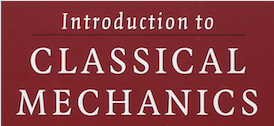# Monthly Archives: December 2017

## 3.35exercise-3-35 Related: Circular Roller Coaster This problem is related to modeling a circular roller coaster with friction. Starting with the substitution $$\omega(\theta)=\dot{\theta}$$$$\ddot{\theta}=\frac{d\omega}{dt}=\frac{d\omega}{d\theta}\frac{d\theta}{dt}=\frac{d\omega}{d\theta}\omega$$ we can eventually reduce the EOM to a first-order differential equation of the form $\dot{\theta}=f(\theta)$. See the exact solution here: Prior, T. and Mele, E. J. (2007). A block slipping on a sphere […]